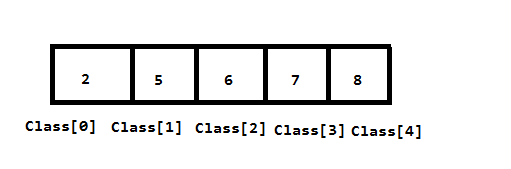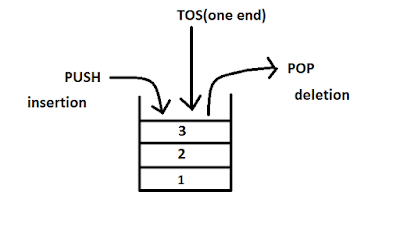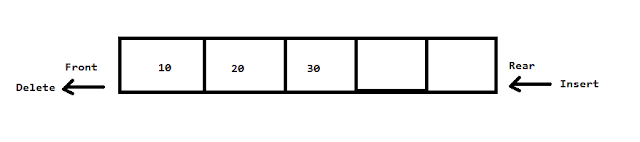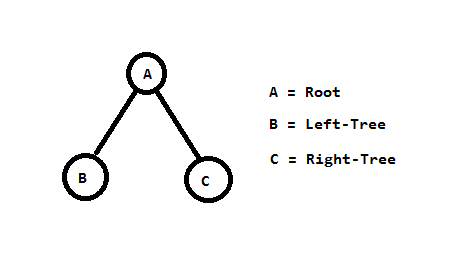What is Data Structure in C || C with Data Structure - Do Tech Blog

Data structure in c is a way of storing data in a computer or device. So that data can be used effectively and easily.

That is, a data structure is a way of storing data so that data can be accessed easily.

## What is Data Structure in C

We all know c language very well. This is a pop (processer oriented programming) based programming language.

The c language has an important role in the data structure.

The type of data structure in c language is followed by something like this -

1. Array
2. Queue
3. Stack
5. Tree

Following this type, the outline of the program is displayed.

## Roll of Data Structure in C

1. How is data saved in memory
2. Which are the ways in which data can be stored
3. How many ways data can be obtained, and can be changed.
4. Knowing the simplest way, the data structure is a way of organizing data.

## 1.Array

The array is a linear data structure. All the data item in an array is stored at the consecutive memory locations of the RAM.

All elements of the array are the same data type. And the name of the array is defined at the time of declarations.

Example - In the following example, there are 5 classes, in which students are shown from the array.In this example, the name of the class array is and the position of the item is from a square bracket. Which starts from zero.

Type of Array

1.Single Dimensional Array

An array containing a liner list of data items. In which the storage of data is only at the memory locations located nearby.

2.Multidimensional-Array

Example of Array

#include <stdio.h>

int main () {

int class ;

printf ("Enter 5 numbers:");

for (int a = 0; a <5; ++ a)

{

scanf ("% d", & class [a]);

}

printf ("display inter number:");

for (int a = 0; a <5; ++ a)

{

printf ("% d \ n", class [a]);

}

return 0;

}

### 2.Stack

A stack is a linear data structure. The stack follows the LIFO approach, ie last in first out or first in last out (FILO). In which whatever last data item is added is at the top of the stack.The stack performs two operations: insertion and deletion operations in which data is inserted and removed.

Recommend: What is Stack in Data Structure (Explain with diagram)

### 3.Queue

The queue is also a linear data structure Queue FIFO (first in first out) or LILO (last in last out)Approach follows.The queue has two ends, front and rear, which contain insert and remove data.

The queue has two operation performances: insertion and deletion

A linked list is a data structure that consists of a collection of nodes. It consists of two nodes. Each node contains information. One node contains data and one contains the address of the next node.

Info is an information or data store in this field.

### 5.TreeThe tree is the data structure in which the collection of nodes is done, the first node of the tree is called the root node and the remaining nodes are divided into sub-trees. Branches connect these nodes.The tree data structure consists of a collection of the root, left sub-tree and right sub-tree.

Normal tree consists of two general tree and binary tree

Recommend: Call By Value And Call By Reference